Examples

Chapter 15 Class 11 Statistics (Term 1)
Serial order wise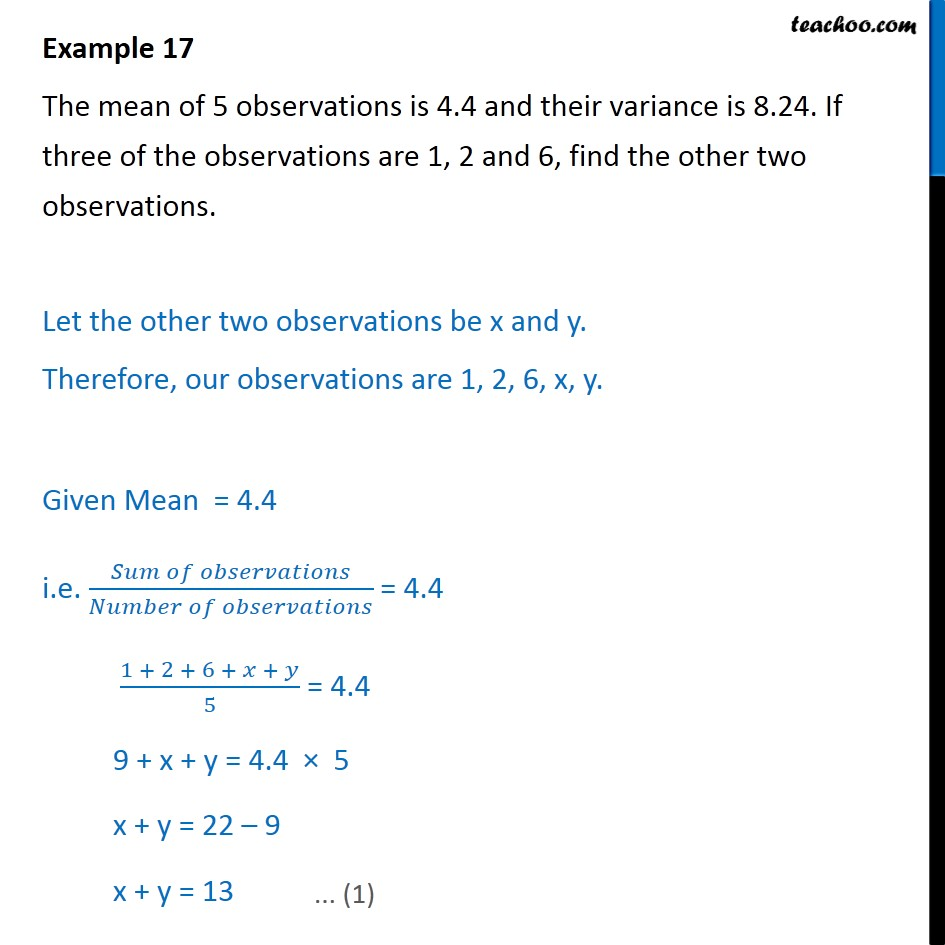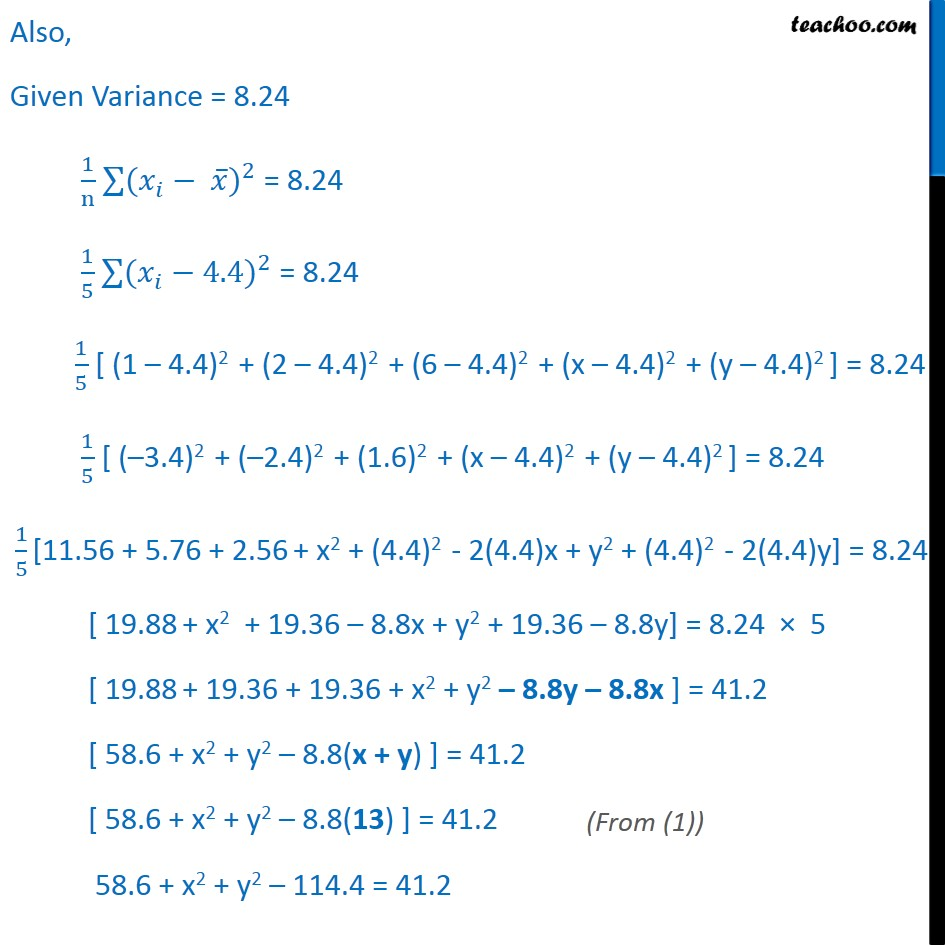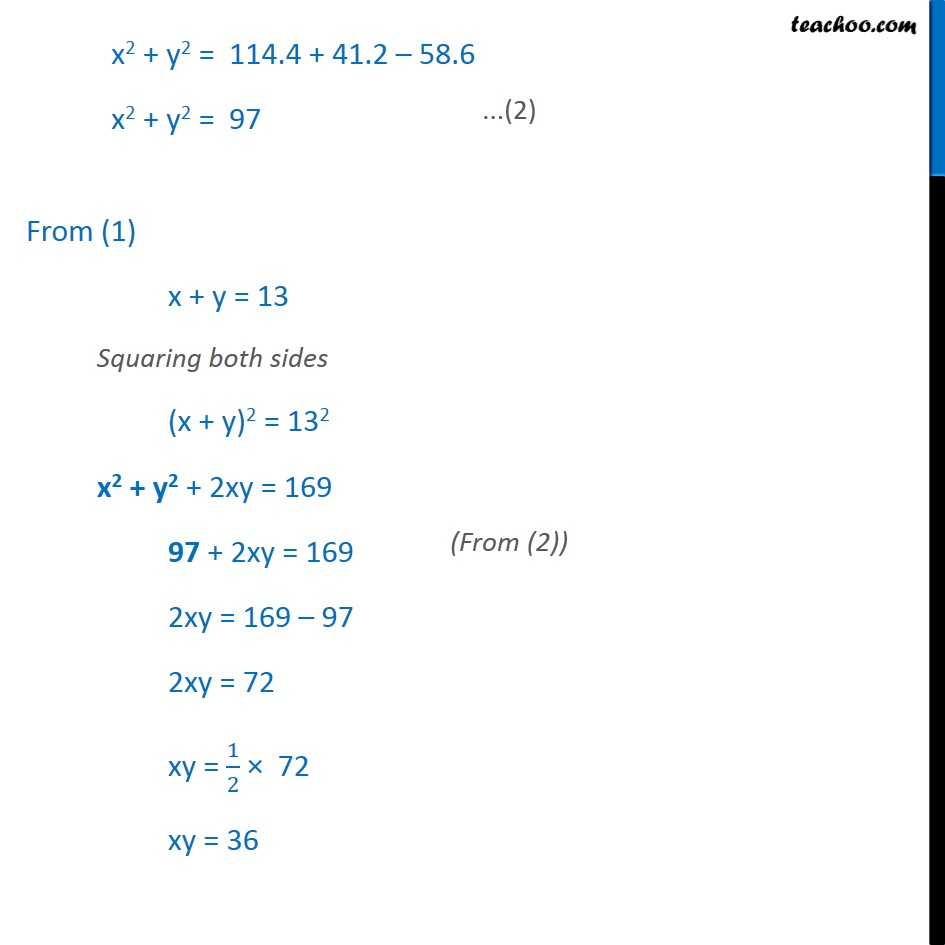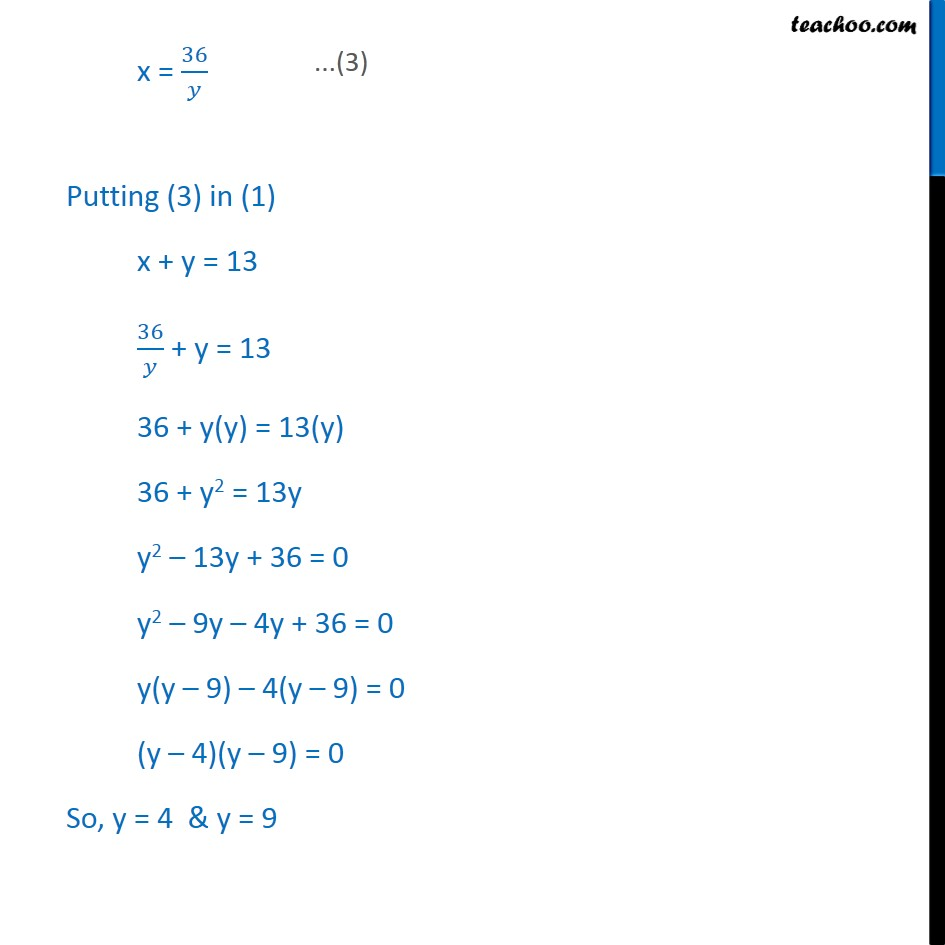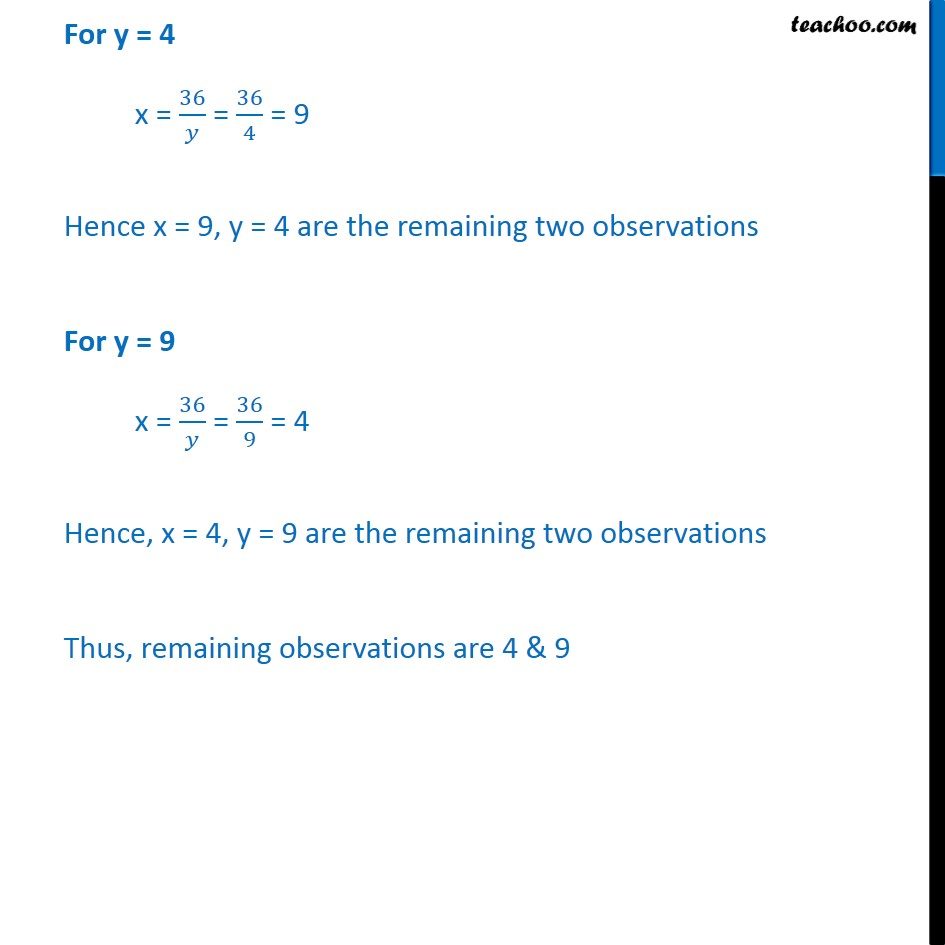### Transcript

Example 17 The mean of 5 observations is 4.4 and their variance is 8.24. If three of the observations are 1, 2 and 6, find the other two observations. Let the other two observations be x and y. Therefore, our observations are 1, 2, 6, x, y. Given Mean = 4.4 i.e. 𝑆𝑢𝑚 𝑜𝑓 𝑜𝑏𝑠𝑒𝑟𝑣𝑎𝑡𝑖𝑜𝑛𝑠﷮𝑁𝑢𝑚𝑏𝑒𝑟 𝑜𝑓 𝑜𝑏𝑠𝑒𝑟𝑣𝑎𝑡𝑖𝑜𝑛𝑠﷯ = 4.4 1 + 2 + 6 + 𝑥 + 𝑦﷮5﷯ = 4.4 9 + x + y = 4.4 × 5 x + y = 22 – 9 x + y = 13 Also, Given Variance = 8.24 1﷮n﷯ ﷮﷮( 𝑥﷮𝑖﷯﷯− 𝑥﷯)﷮2﷯ = 8.24 1﷮5﷯ ﷮﷮( 𝑥﷮𝑖﷯﷯−4.4)﷮2﷯ = 8.24 1﷮5﷯ [ (1 – 4.4)2 + (2 – 4.4)2 + (6 – 4.4)2 + (x – 4.4)2 + (y – 4.4)2 ] = 8.24 1﷮5﷯ [ (–3.4)2 + (–2.4)2 + (1.6)2 + (x – 4.4)2 + (y – 4.4)2 ] = 8.24 1﷮5﷯ [11.56 + 5.76 + 2.56 + x2 + (4.4)2 - 2(4.4)x + y2 + (4.4)2 - 2(4.4)y] = 8.24 [ 19.88 + x2 + 19.36 – 8.8x + y2 + 19.36 – 8.8y] = 8.24 × 5 [ 19.88 + 19.36 + 19.36 + x2 + y2 – 8.8y – 8.8x ] = 41.2 [ 58.6 + x2 + y2 – 8.8(x + y) ] = 41.2 [ 58.6 + x2 + y2 – 8.8(13) ] = 41.2 58.6 + x2 + y2 – 114.4 = 41.2 x2 + y2 = 114.4 + 41.2 – 58.6 x2 + y2 = 97 From (1) x + y = 13 Squaring both sides (x + y)2 = 132 x2 + y2 + 2xy = 169 97 + 2xy = 169 2xy = 169 – 97 2xy = 72 xy = 1﷮2﷯ × 72 xy = 36 x = 36﷮𝑦﷯ Putting (3) in (1) x + y = 13 36﷮𝑦﷯ + y = 13 36 + y(y) = 13(y) 36 + y2 = 13y y2 – 13y + 36 = 0 y2 – 9y – 4y + 36 = 0 y(y – 9) – 4(y – 9) = 0 (y – 4)(y – 9) = 0 So, y = 4 & y = 9 For y = 4 x = 36﷮𝑦﷯ = 36﷮4﷯ = 9 Hence x = 9, y = 4 are the remaining two observations For y = 9 x = 36﷮𝑦﷯ = 36﷮9﷯ = 4 Hence, x = 4, y = 9 are the remaining two observations Thus, remaining observations are 4 & 9